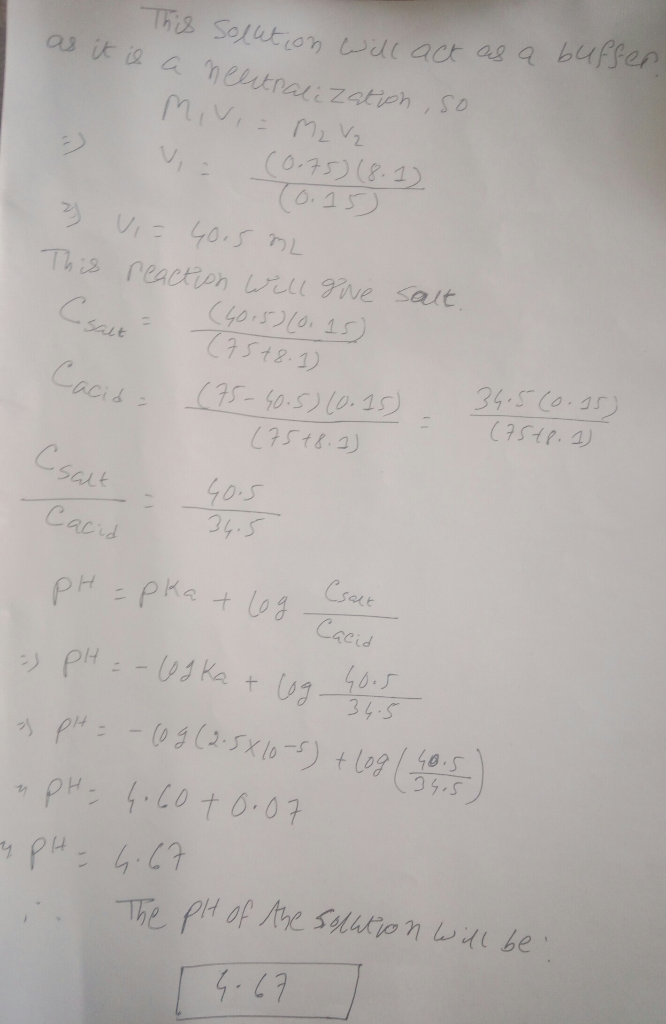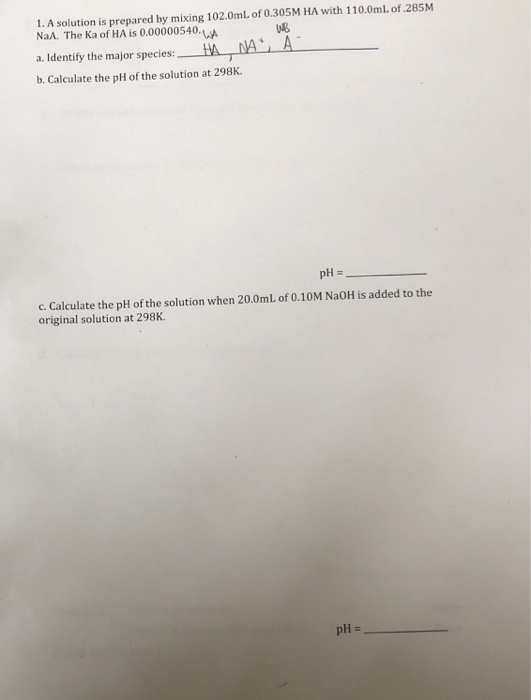Question

# Calculate the pH of a solution made by mixing 75.0mL of 0.15M HA (Ka= 2.5x10-5) with...

Calculate the pH of a solution made by mixing 75.0mL of 0.15M HA (Ka= 2.5x10-5) with 8.1mL of 0.75M NaOH#### Earn Coins

Coins can be redeemed for fabulous gifts.

Similar Homework Help Questions
• ### 1. Determine the pH of a buffer solution prepared by mixing 25.3mL of 0.35M HA (Ka=...

1. Determine the pH of a buffer solution prepared by mixing 25.3mL of 0.35M HA (Ka= 1.6x10-5) with 15.3mL of 0.45M NaA. 2.100 mL of buffer solution that is 0.15M HA (Ka= 6.8x10-5) and 0.20M NaA is mixed with 17.3mL of 0.25M HCl. What is the pH of the resulting solution? 3. Calculate the pH of a solution made by mixing 75.0mL of 0.15M HA (Ka= 2.5x10-5) with 7.5mL of 0.75M NaOH 4. 100 mL of buffer solution that is...

• ### Calculate the pH of a solution made by mixing 14.5mL of 0.15M HCl with 5.3mL of...

Calculate the pH of a solution made by mixing 14.5mL of 0.15M HCl with 5.3mL of 0.10M NaOH. Enter your answer to two places after the decimal.

• ### Calculate the pH for each of the following conditions: a. 0.15M acetic acid b. What is...

Calculate the pH for each of the following conditions: a. 0.15M acetic acid b. What is the pH of the resulting solution if 30.00 mL of 0.15M acetic acid is added to 10.00 mL of 0.15 M NaOH? Assume that the volumes of solutions are additive, Ka = 1.8*10^-5 for CH3CO2H c. What is the pH of a solution made by mixing 30.00 mL of 0.15M acetic acid with 30.00 mL oof 0.15M KOH? Assume that the volumes of the...

• ### 100 mL of buffer solution that is 0.15M HA (Ka= 6.8x10-5) and 0.20M NaA is mixed...

100 mL of buffer solution that is 0.15M HA (Ka= 6.8x10-5) and 0.20M NaA is mixed with 18.2mL of 0.35M NaOH. What is the pH of the resulting solution?

• ### 100 mL of buffer solution that is 0.15M HA (Ka= 6.8x10-5) and 0.20M NaA is mixed...

100 mL of buffer solution that is 0.15M HA (Ka= 6.8x10-5) and 0.20M NaA is mixed with 29.7mL of 0.25M HCl. What is the pH of the resulting solution?

• ### Determine the pH of a buffer solution prepared by mixing 11.4mL of 0.35M HA (Ka= 1.6x10-5)...

Determine the pH of a buffer solution prepared by mixing 11.4mL of 0.35M HA (Ka= 1.6x10-5) with 25mL of 0.45M NaA.

• ### Calculate the pH of the solution that results from each mixture. a. 50.0mL of 0.15M HCHO2...

Calculate the pH of the solution that results from each mixture. a. 50.0mL of 0.15M HCHO2 with 75.0mL of 0.13M NaCHO2 b.125.0mL of 0.10M NH3 with 250.0mL of 0.10M NH4CL

• ### 1. A solution is prepared by mixing 102.0mL of 0.305M HA with 110.0mL of.285M NaA. The...1. A solution is prepared by mixing 102.0mL of 0.305M HA with 110.0mL of.285M NaA. The Ka of HA is 0.00000540. B a. Identify the major species: HAMA A b. Calculate the pH of the solution at 298K. pH = c. Calculate the pH of the solution when 20.0mL of 0.10M NaOH is added to the original solution at 298K. pH =

• ### Calculate the pH during titration of 75.0mL of 0.100M benzoic acid by 0.100M NaOH before the...

Calculate the pH during titration of 75.0mL of 0.100M benzoic acid by 0.100M NaOH before the addition of NaOH and after 20mL NaOH are added. Ka=6.3x10^-5

• ### 1. a)Calculate the pH of a 0.30M formic acid solution (Ka=1.8*10^-4)Weak monoprotic acid. b)Calculate the Ka...

1. a)Calculate the pH of a 0.30M formic acid solution (Ka=1.8*10^-4)Weak monoprotic acid. b)Calculate the Ka for a 0.050M solution of HA (weak avid if the pH=4.65 c)What is the pH of the solution which results from mixing 50.0mL of 0.30M HF (aq) and 50.0mL of 0.30M NaOH (aq) at 25C? (Kb of F- =1.4*10^-11) I am having a hard time with these so as much detail as possible would be great, thank you for your time and your help.